## Posts

Showing posts from February 27, 2022

### Constructing an Inscribed Circle: for GCSE, IGCSE and GCE O-Level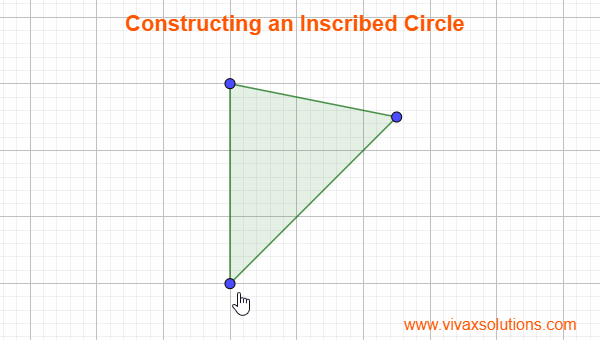Inscribed Circle A circle that touches the three sides of a triangle from inside is called  an inscribed circle. In order to construct an inscribed circle, Construct the angular bisector of each angle Note the point where the three angular bisectors intersect at Use the point in step 2, as the centre, use the compasses to draw the circle;  it will touch all three sides, as if each side was a tangent For the construction of a circumcircle , please follow this link .

### Constructions and Loci for GCSE and IGCSE Maths; circumcircle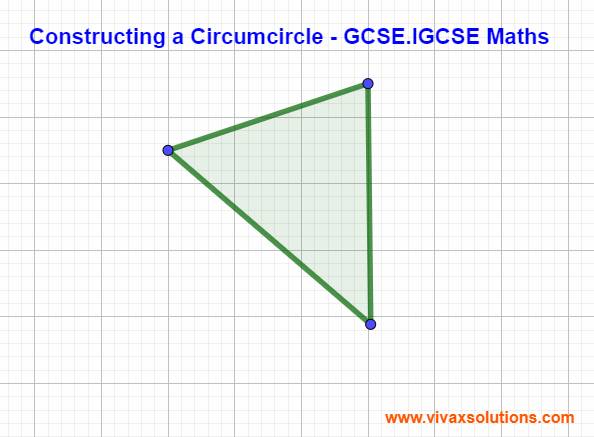A circle that touches the vertices of a tringle from outside is called a circumcircle. In order to construct it, the following simple steps can be followed in order: Construct the three perpendicular lines of each side. Find the point where the lines in step 1 intersect.  Using the point in step 2) as the centre. just complete the circle with your compasses. It is the circumcircle.

### Perpendicular Lines - for GCSE, IGCSE, O Level & AS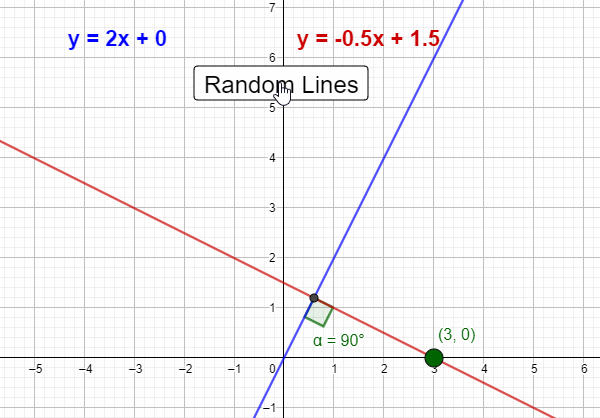If two lines are perpendicular, the gradient of one line is the negative reciprocal of the other line. E.g.1 Find the equation of the perpendicular line to the equation, y = 2x - 3 that goes through the point (4,5). The gradient of the perpendicular line = -1/2 = -0.5 For the perpendicular line, m = -0.5 x = 4, y = 5 y = mx + c 5 = -0.5(4) + c c = 7 y = -0.5x + 7 The equation of the perpendicular line, y = -0.5x + 7 E.g.2 Find the equation of the perpendicular line to the equation, y = 1/2x - 5 that goes through the point (2,-1). The gradient of the perpendicular line = -1/(1/2) = -2 For the perpendicular line, m = -2 x = 2, y = -1 y = mx + c -2 = -2(2) + c c = 2 y = -2x + 2 The equation of the perpendicular line, y = -2x + 2 E.g.3 Find the equation of the perpendicular line to the equation, y = -4x - 3 that goes through the point (8,1). The gradient of the perpendicular line = -1/(-4) = 1/4 For the perpendicular line, m = 1/4 x = 8, y = 1 y = mx + c 1 = 0.25(8) + c c = -1 y = -1/4x

### Measuring the distance to stars for A Level physics - parsec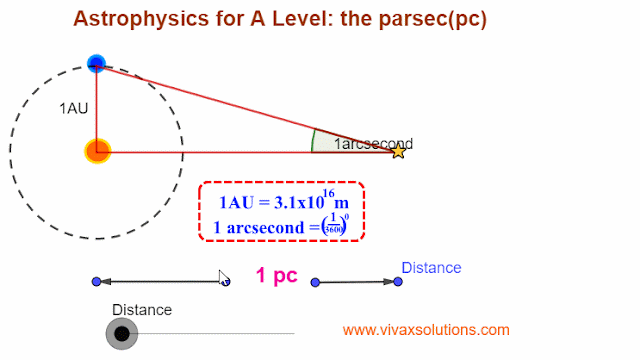When it comes to distances involved in the objects in space, three major units are used. They are, astronomical unit light-year parsec Astronomical unit - AU This is the average distance between the Sun and the Earth. 1AU = 150 million km = 1.5 x 10 11 m The astronomical unit, AU,  is used to express the distance between the Earth and the other planets in our solar system. Light-year - ly   The distance travelled by light in one year is called a light-year. 1 light-year = 3 x 10 8 x 3600 x 24 x 365 = 9.46 x 10 15 m parsec -pc The distance at which 1AU subtends an angle of 1 arcsecond is called a parsec. 1 arcsecond - (1/3600)° tan (1 arcsecond) = 1AU/1pc 1 pc =  1AU x tan(1 arcsecond) 1pc = 1.5 x 10 11 x tan(1 arcsecond) 1pc = 3.1 x 10 16 m or 1 pc = 3.26 ly You can calculate the angle in arcseconds and pc with the following simulation:

### Total resistance of combined resistors - series and parallel circuits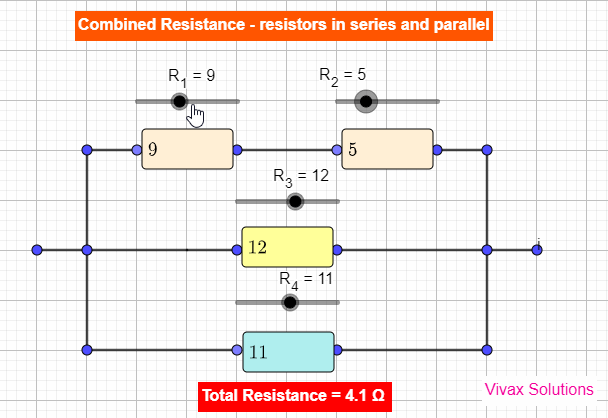Resistors in series and parallel Resistors can be combined in two different methods; they are in series and in parallel. If two resistors, R 1 and R 2 2 , are in series, the total resistance, R t , is given by the following formula. R t = R 1 + R 2 If the same resistors are in parallel, the total resistance is as follows: R t = 1/R 1 + 1/R 2 E.g. 1 Two resistors, 6 and 3 are connected in series. Find the total resistance. R t = R 1 + R 2 R t = 6 + 3 R t = 9 E.g.2 Two resistors, 10 and 15 are in parallel. Find the total resistance.  1/R t = 1/10 + 1/15 1/R t = 5/30 R t = 6 If resistors are in series The total resistance is bigger than the highest individual resistance of the circuit. The current through each resistor is the same. The total voltage splits up across each resistor. If one resistors is removed, the currents does not flow through the entire circuit E.g. Christmas tree decorating lights If resistors are in parallel The total resistance is smaller than t

### Finding area under curves by integration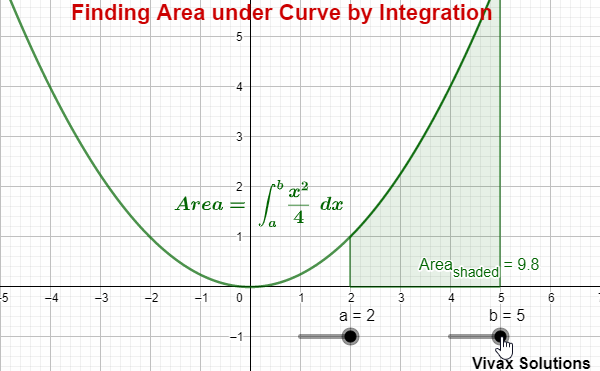The area under a curve and the x-axis can be found by integrating the curve between two chosen values of x. Area = ∫ x 2 /4 dx E.g. Area under the curve between x = 1 and x = 4 = ∫ x 2 /4 dx = [x 3 /12]  4 1 = 64/12 - 1/12 = 63/12 = 5.3(1 d.p.) For interactive practice of the above, here is the applet: For a more comprehensive tutorial on integration, including interactive practice, please follow the link :

### Resistance of a rectangular block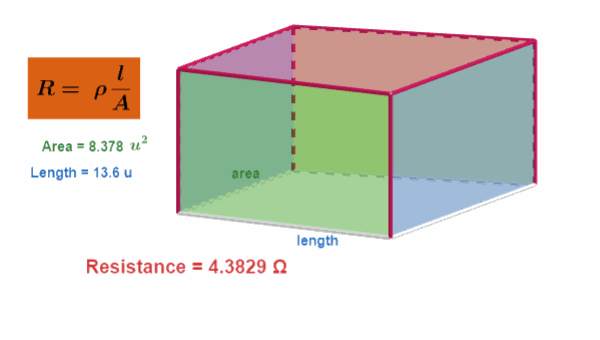The resistance of a block/wire depends on two factors: they are the cross sectional area and the length. The relationship is given by the following formula: R = ρ l/A The longer the wire/block, the greater the resistance. The thinner the wire/block, the greater the resistance. The constant, ρ, in this case is called resistivity. It depends only on the substance. E.g. Metal Resistivity - Ωm Silver 1.59x10 -8 Nickel 6.99x10 -7 Iron 1.0x10 -7 Copper 1.68x10 -8 Lead 2.2x10 -7 Tungsten 5.69x10 -8       E.g.1 The dimensions of a metal cuboid are 6cm, 4cm and 2cm respectively. Find its resistance, if the resistivity is 1.2 X 10 -8 Ωm and the current enters through the smallest surface. R = ρl/A A = 8 x 10 -4 m 2 l= 6 x 10 -2 m R = 1.2 X 10 -8 x 6 x 10 -2 / 8 x 10 -4 R = 9 X 10 -7 Ω E.g.2 The radius of a wire is 2cm and the length is 8cm. If the resistivity is 3x10 -6 , find the resistance of the wire. A = πr 2 = 3.142*2 2 *10 -4 = 12.562*10 -4 R = ρl